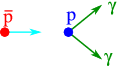# 9.2: Invariant Mass

$$\newcommand{\vecs}{\overset { \rightharpoonup} {\mathbf{#1}} }$$ $$\newcommand{\vecd}{\overset{-\!-\!\rightharpoonup}{\vphantom{a}\smash {#1}}}$$$$\newcommand{\id}{\mathrm{id}}$$ $$\newcommand{\Span}{\mathrm{span}}$$ $$\newcommand{\kernel}{\mathrm{null}\,}$$ $$\newcommand{\range}{\mathrm{range}\,}$$ $$\newcommand{\RealPart}{\mathrm{Re}}$$ $$\newcommand{\ImaginaryPart}{\mathrm{Im}}$$ $$\newcommand{\Argument}{\mathrm{Arg}}$$ $$\newcommand{\norm}{\| #1 \|}$$ $$\newcommand{\inner}{\langle #1, #2 \rangle}$$ $$\newcommand{\Span}{\mathrm{span}}$$ $$\newcommand{\id}{\mathrm{id}}$$ $$\newcommand{\Span}{\mathrm{span}}$$ $$\newcommand{\kernel}{\mathrm{null}\,}$$ $$\newcommand{\range}{\mathrm{range}\,}$$ $$\newcommand{\RealPart}{\mathrm{Re}}$$ $$\newcommand{\ImaginaryPart}{\mathrm{Im}}$$ $$\newcommand{\Argument}{\mathrm{Arg}}$$ $$\newcommand{\norm}{\| #1 \|}$$ $$\newcommand{\inner}{\langle #1, #2 \rangle}$$ $$\newcommand{\Span}{\mathrm{span}}$$$$\newcommand{\AA}{\unicode[.8,0]{x212B}}$$

One of the key numbers we can extract from mass and momentum is the invariant mass, a number independent of the Lorentz frame we are in

$W^2c^4 = (\sum_i E_i)^2 - (\sum_i {\vec{p}}_i)^2 c^2.$

This quantity takes it most transparent form in the center-of-mass, where $$\sum_i {\vec{p}}_i = 0$$. In that case

$W = E_{\mathrm{CM}}/c^2,$

and is thus, apart from the factor $$1/c^2$$, nothing but the energy in the CM frame. For a single particle $$W=m_0$$, the rest mass.

Most considerations about processes in high energy physics are greatly simplified by concentrating on the invariant mass. This removes the Lorentz-frame dependence of writing four momenta. I

As an example we look at the collision of a proton and an antiproton at rest, where we produce two quanta of electromagnetic radiation ($$\gamma$$’s), see Figure $$\PageIndex{1}$$, where the anti proton has three-momentum $$(p,0,0)$$, and the proton is at rest.Figure $$\PageIndex{1}$$: A sketch of a collision between a proton with velocity $$v$$ and an antiproton at rest producing two $$\gamma$$ quanta.

The four-momenta are

\begin{aligned} p_{\mathrm{p}} &=& (p_{\mathrm{lab}},0,0,\sqrt{m_p^2 c^4+ p_{\mathrm{lab}}^2 c^2})\nonumber\\ p_{\bar{\mathrm{p}}} &=& (0,0,0,m_p c^2).\end{aligned}

From this we find the invariant mass

$W = \sqrt{2 m_p^2 + 2m_p\sqrt{m_p^2+ p_{\mathrm{lab}}^2 /c^2}}$

If the initial momentum is much larger than $$m_p$$, more accurately

$p_{\mathrm{lab}} \gg m_p c,$

we find that

$W \approx \sqrt{2 m_p p_{\mathrm{lab}}/c},$

which energy needs to be shared between the two photons, in equal parts. We could also have chosen to work in the CM frame, where the calculations get a lot easier.

This page titled 9.2: Invariant Mass is shared under a CC BY-NC-SA 2.0 license and was authored, remixed, and/or curated by Niels Walet via source content that was edited to the style and standards of the LibreTexts platform; a detailed edit history is available upon request.